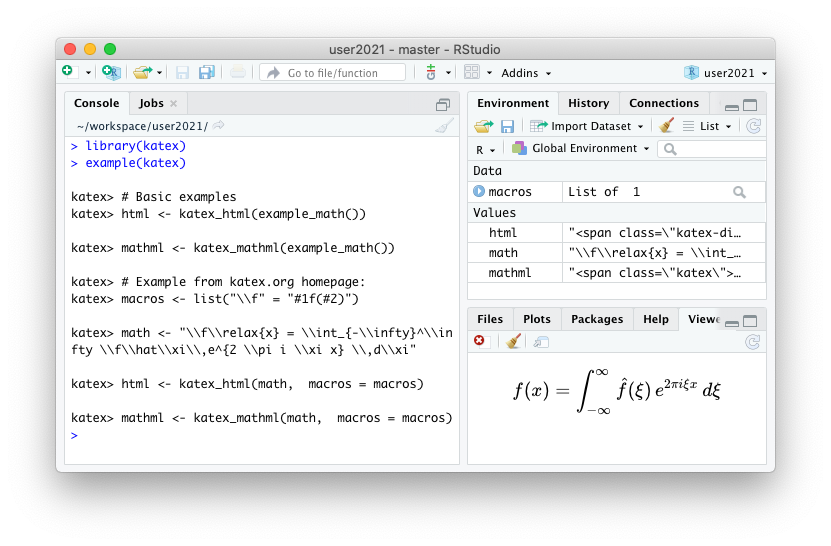rOpenSci | New package katex: rendering math to HTML and MathML in R# New package katex: rendering math to HTML and MathML in R

A new rOpenSci package katex is now on CRAN. This package allows for converting latex math expressions to HTML and MathML for use in markdown documents or package documentation.

The R package uses the katex javascript library, but the rendering is done directly in R using the V8 engine (i.e. server-side), which eliminates the need for embedding the MathJax library into html pages.

## 🔗 What it means to render server-side

To display a tex math in html we need a math typesetting library. The standard solution is to include MathJax with the html page, which attempts to convert math to html in the browser, upon loading of the webpage. However MathJax is quite heavy, which slows down the page, and it may not work in browsers where use of JavaScript or external libraries is restricted.

An alternative solution is to perform the rendering when you generate the html page, and embed the pre-generated html snippet directly in the document. This makes the html file slightly larger, but the page loads much faster and is more robust because we do not need a complex dependency on the client. This is often called “server-side”, because usually html is generated by a web server.

The new katex package provides server-side math rendering in R. It can be used to include math when generating html in R (e.g. via Rmarkdown or R package documentation) without having to include MathJax in the output html document.

## 🔗 Tex math rendering in R

Functions katex_html() and katex_mathml() render a string with math to a html and mathml string. The html output has an option preview which will show a preview of the generated HTML snippet directly in a browser or the RStudio viewer.

# Basic example: render and show math html
library(katex)
math <- example_math()
html <- katex_html(math, preview = TRUE)

# Convert to mathml
mathml <- katex_mathml(math)
cat(mathml)Note that by default, katex_html actually returns a mix of HTML for visual rendering and includes MathML for accessibility. The katex docs site has more detailed information and additional options. Hopefully this can be used to enable support for server-side math in knitr/rmarkdown soon!

## 🔗 Embedding math in R documentation

The katex package also has a powerful helper function math_to_rd to insert math into R documentation (.rd) files. This uses Katex rendering for R documentation rendered in html format, and the appropriate latex macros for documentation rendered in pdf or plain-text.

You can call this directly from within your roxygen2 or Rd files using the \Sexpr macro like this:

\Sexpr[results=rd, stage=build]{
katex::math_to_rd(katex::example_math())
}

$f(x)= {\frac{1}{\sigma\sqrt{2\pi}}}e^{- {\frac {1}{2}} (\frac {x-\mu}{\sigma})^2}$

Check out the ?math_to_rd manual page in R or the math_to_rd docs for live examples and more details.

Filed under
Share
Suggest an edit Open a pull request
Browse